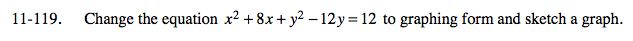### Home > A2C > Chapter 11 > Lesson 11.2.6 > Problem11-119

11-119.Complete the squares for both x and y.

(x2 + 8x + 16) + (y2 − 12y + 36) = 12 + 16 + 36

(x + 4)2 + (y − 6)2 = 64

This is the equation of a circle whose center is at (−4, 6) and whose radius is 8.